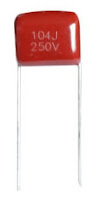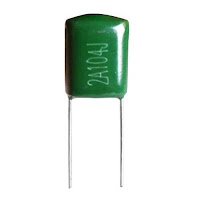## Friday, July 9, 2021Fig. 1 - Various capacitors - how to read correctly

Due to many manufacturers and various norms and standards established nowadays, many acronyms are implemented in electronic components and use a wide variety of codes to describe their characteristics, which makes them difficult to read, there is an intrinsic coding to indicate the capacitors values, and manufacturers use different methods.

Sometimes generating a bit of confusion, some indications such as; tolerance and also the working supply voltage often aren't clearly written on them.

Will explain how to read the capacitors, identifying: microfarads (μF), nanofarards (nF), picofarads (pF), tolerance, voltage, and so on.

For values ​​equal greater than 1000nF (eg with aluminum or tantalum electrolytics), they mostly write the value on the body followed by the abbreviation for microfarad (μF).

For values ​​less than 1μF (1 microfarad), the issue is not so clear.
Generally, an encoding consisting of a three-digit number followed by a letter is used.

Before the most skeptics and purists come to question this Post, let us clarify that the correct abbreviation for microfarad is the Greek symbol; micro (μ). Which is a prefix of the International System of Units denoting a factor of 10−6 (one millionth).

Confirmed in 1960, the prefix comes from the Greek; μικρός (transliterated: mikros), meaning small. Followed by the capital letter F.

Usually when we're doing component descriptions, we don't always have the Greek symbols available on our keyboard, so to prevent this symbol from being wrongly transcribed, we substitute it for the lowercase letter "u", although we mustn't forget that we're always talking about the letter. "μ" (micro).

We have other cases, examples of this type, it is the symbol Ω (ohm) that is sometimes replaced by the letter "R" or, in some other cases nothing is written.

As mentioned at the beginning, with the exception of electrolytic capacitors that generally far exceed the value of 1 microfarad, the universe of capacitors used in electronics consists of capacitors with values ​​ranging from a few pF or picofarad (ceramic or disk capacitors look like lentils) to those close to 1 microfarad or 1μF (multi-layer polyester).

Before continuing, it is worth remembering "for whoever forgot" the subject of submultiples.

## Submultiples

A pF (picofarad) is the smallest submultiple that exists to "practically" indicate capacity. I say practical because there are still smaller submultiples, SI Prefixes (International System of Units)

(deci, centi, milli, micro, nano, pico, femto, atto, zepto and yocto), but they are not used in electronics. 1 picofarad is 1,000,000 (1 million) times less than 1 microfarad (μF).

Halfway between picofarad and microfarad there is another sub-multiple called nanofarad widely used and it is 1000 times larger than 1 picofarad and 1000 times smaller than 1 microfarad.

## Typical Capacitor Values

For capacitors facing between 1pF to 1μF (almost all capacitors except for electrolytic), reference values ​​are indicated with a three-digit number followed by a letter.

The first two digits indicate the starting number, while the third digit represents the number of zeros that must be added to the starting number to get the ending value.

The result obtained is necessary to consider it in picofarad.

## Examples of encodings

Let's use it as an example; 4 types of captions written on the capacitors, as shown in Figure 3 below.

In the capacitor in Figure 2, we can see in the description only a set of three numbers "104", which representing the capacitance in Picofarad reading.Figure 2 - Capacitor with only capacitance captions

104 - Which is its capacitance in pF, and without any further information.

The capacitor in Figure 3, we can see in the description the set of 3 numbers "400" which representing the working voltage, followed by the letter "V", which is the working voltage indication, and the set of three numbers below "104", which represents the reading in Picofarad.Figure 3 - Capacitor with voltage and capacitance value captions

400V - Which is the working voltage.

104 - What is its value in pF

The capacitor in Figure 4, we can see in the description the set of 3 numbers "104", which represents the reading in Picofarad, followed by the letter "J", representing Tolerance, and the set of three numbers "250" represent the working voltage followed by the letter "V", which is the working voltage indication.Figure 4 - Capacitor with capacitance, tolerance, voltage captions

104 - What is your capacitance in pF

J - It's the tolerance

250V - Is the working voltage.

The capacitor in Figure 5, we can see that in the description it starts with a number and a letter "2A" which represents the value of the maximum working voltage, then the set of 3 numbers "104", which represents the reading in Picofarad, followed by the letter "J" representing Tolerance.Figure 5 - Capacitor with maximum voltage, capacitance, tolerance

2A - Which is the value of your maximum voltage

104 - What is your capacitance in pF

## Let's Practice:

Let's say you have a capacitor with the nomenclature written "472", just as we take resistor readings, the third capacitor digit is also the multiplier, which means it would be: 47 + 2 zeros, which means 4700 pF (picofarad).

So if we exceed 1000 picofarad, we can use Sub-multiples, "like we do with meters/kilometers". As already clarified above that:

1μF = 1000nF
1nF = 1000pF

So, we can say that our 4700pF capacitor is 4.7nF.

In this case, it is not convenient to use the micro unit because the value would not be easy to read (0.0047μF).

With larger values, such as used capacitor filters number 104, that is, 10 + 4 = 100,000 pF or also 100nF, it is common for manufacturers to use the nomenclatures written on the capacitor body 0.1μF or .1μf (point one μF) .

## Practical reading of the Polyester Capacitor

100nF Capacitor, tolerance of  ± 5% and maximum working voltage of 100V, Figure 5 above.

In this capacitor we have 6 alphanumeric digits, 2A104J.

• The first two initial 2A digits refer to Maximum Voltage, we can use the complete EIA table codes that indicate the maximum capacitors work voltages in direct voltage (DC).

EIA Table of Code Indicators of Working Voltages of a Capacitor

 0G = 4VDC 0L = 5.5VDC 0J = 6.3VDC 1A = 10VDC 1C = 16VDC 1E = 25VDC 1H = 50VDC 1J = 63VDC 1K = 80VDC 2A = 100VDC 2Q = 110VDC 2B = 125VDC 2C = 160VDC 2Z = 180VDC 2D = 200VDC 2P = 220VDC 2E = 250VDC 2F = 315VDC 2V = 350VDC 2G = 400VDC 2W = 450VDC 2H = 500VDC 2J = 630VDC 3A = 1000VDC
• The next three digits refer to its capacitance, in the case as already exemplified 104 = 10 + 4 zeros, which is equal to 100,000pF = 100nF.

• The last digit is the Letter "J", right after the three digits, determines the tolerance of the component.

It is interesting to note the fact that some letters correspond to "asymmetric tolerances", such as "P", that is, the component may have a capacity greater than indicated, but not less.

This type of tolerance is used with "filter" capacitors, where a value possibly higher than indicated does not minimize circuit operation, as we can see in the EIA table below.

## EIA Table of Code Working Tolerance Indicators of a Capacitor

• B = ± 0.10pF
• C = ± 0.25pF
• D = ± 0.5pF
• E = ± 0.5%
• F = ± 1%
• G = ± 2%
• H = ± 3%
• J = ± 5%
• K = ± 10%
• M = ± 20%
• N = ± 30%
• P = ± +100%, - 0%
• Z = ± +80%, - 20%
In the vast majority of cases, it may be useful to know the exact maximum voltage the capacitor can withstand without bursting or damaging its internal properties.

As we know, a capacitor is made up of a series of metal plates insulated from each other. This insulating material is very subtle, especially in the case of high-value capacitors.

On the other hand, if the voltage is too high, there is a risk that an electrical arc will pass through the electrical insulation between the plates, breaking it and shorting the capacitor.

For this reason, the insulating material used is designed to work up to a certain maximum voltage level, so let's look at these capacitor voltages.

## Dimensions of a Voltage-Based Capacitor

Often the maximum working voltage can be found clearly written, especially on capacitors designed to work with high voltages, other times the voltage value is not directly indicated.

It often happens with capacitors used in low voltage circuits. These capacitors support voltages between 50V and 100V, well above the typical working voltages of 5V, 12V, 18V, 24V, 48V.

A super important tip when designing or analyzing a circuit and not knowing for sure the capacitor working voltage, is to take into account the size, which in this case "size is important", as we cannot work with the structure of a capacitor.

A high voltage and small size, of course there are exceptions, tantalum capacitors are altogether quite small compared to their capacitance, but as I said, "compared to their capacitance, not their voltage".

Last but not least, there is a numeric encoding used by some manufacturers which consists of a number followed by a letter. In the table of tolerances we can see the maximum working voltages.

As with everything related to technology, nothing is absolute and therefore a component manufacturer always appears, which uses systems to indicate values ​​different from those we describe. In any case, in general terms, this article's description fits very well (sometimes with slight variations) to most commercial capacitors nowadays.

If you have any questions, suggestions or corrections, please leave them in the comments and we will answer them soon.